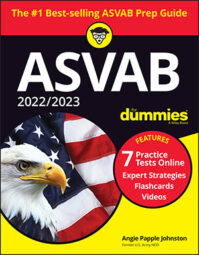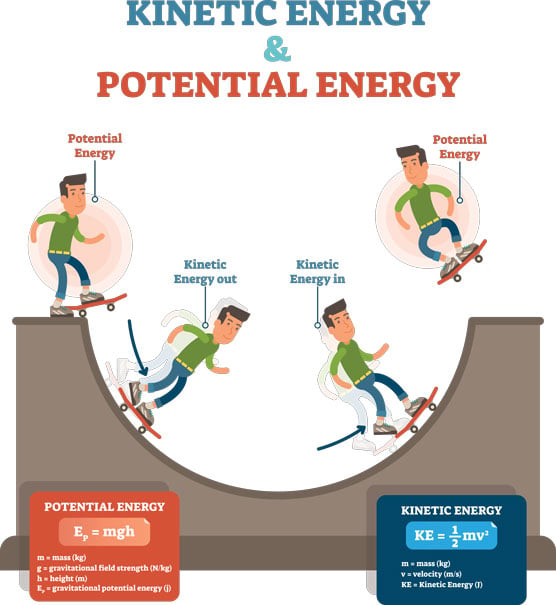##### 2022 / 2023 ASVAB For DummiesOn the Mechanical Comprehension subtest of the ASVAB test, you need to know the definition of work and understand the basics of potential and kinetic energy and resistance.

Mechanically speaking, work happens when a force (usually measured in pounds) moving over a measurable distance (usually measured in feet) overcomes a resistance. In the United States, the unit of measure for work is often called a foot-pound. (Note: The rest of the world uses the newton-meter, or joule.) One foot-pound of work occurs when a 1-pound weight is lifted to a height of 1 foot. You can represent this concept in equation form:

Work = Force × Distance
Work is different from effort; work is the result of effort. You can think of effort as being force and of work as being what you produce with that force.

## Working out the difference between potential and kinetic energy

Energy is the capacity to do work. Every object in the universe has energy, and it’s either potential or kinetic. Potential energy is stored energy — energy that’s not doing anything at the moment but that’s in the object by virtue of its position in a field. If a book is resting in your hands, the book itself is holding potential energy. If you raise the book over your head, you’re increasing its potential energy (thanks to the Earth’s gravitational pull). When you accidentally drop it, all its potential energy becomes kinetic energy, or energy in motion. When the book hits the ground, its energy becomes potential again.©VectorMine/Shutterstock.com

Potential energy can’t be transferred between objects. The more massive an object is, the more potential and kinetic energy it has (so a bowling ball contains more energy than a basketball does). Both these forms of energy are measured in joules.

## Overcoming resistance

The resistance that the work overcomes isn’t the same thing as the weight of the object. (If you’ve ever tried to put your freaked-out cat in a cat carrier to go to the vet, you know what I mean.) In other words, if you try to move a 1,200-pound piano, you’ll probably notice a measurable difference between the amount of work it takes to shove it along the floor and the amount of work it takes to carry it up the stairs. But don’t take my word for it — you can demonstrate this concept at home. First, find a 1,200-pound piano and push it across the floor. Next, put it on your back and carry it up the stairs. See the difference? (Really, don’t put the piano on your back. I’m just trying to make a point here.)

When you move the piano across the floor, you’re really working (pushing) against the frictional resistance (the force that’s produced when two surfaces rub together) of the piano rather than its full weight. Under these circumstances, the frictional resistance of the piano offers less resistance than its full weight. There are times when an object’s full weight is less than its frictional resistance. Consider trying to push a textbook across a deep-pile carpet. Picking the book up and carrying it is easier.

## Gaining power by working more quickly

Power is the rate of work. If Mary Lou is able to lift more 50-pound sacks of potatoes onto the truck bed in 10 minutes than Joe is, Mary Lou is more powerful than Joe. Mathematically speaking, Power = Work / Time.

In this formula, work is usually measured in foot-pounds, time is measured in minutes, and power is measured in foot-pounds per minute. However, the unit of measure for power is commonly put in terms of horsepower (hp).

Horsepower is derived from the estimate that an average horse can do 33,000 foot-pounds of work in 1 minute (according to James Watt). Therefore, 1 horsepower = 33,000 foot-pounds per minute. One horsepower is also the same as 550 foot-pounds per second.